# NCERT Solutions for Class 10 Maths Chapter 13 Surface Areas and Volumes Ex 13.1

NCERT Solutions for Class 10 Maths Chapter 13 Surface Areas and Volumes Ex 13.1 are part of NCERT Solutions for Class 10 Maths. Here are we have given Chapter 13 Surface Areas and Volumes Class 10 NCERT Solutions Ex 13.1.

Students find NCERT Solutions for Class 10 Maths Chapter 13 Surface Areas and Volumes quite helpful for their CBSE Board Preparation. Refer to our Textbook Solutions any time, while doing your homework or while preparing for the exam. All questions and answers from the NCERT Book of class 10 Math Chapter 13 are provided here for you for free.

 Board CBSE Textbook NCERT Class Class 10 Subject Maths Chapter Chapter 13 Chapter Name Surface Areas and Volumes Exercise Ex 13.1 Number of Questions Solved 9 Category NCERT Solutions

## NCERT Solutions for Class 10 Maths Chapter 13 Surface Areas and Volumes Ex 13.1

Question 1.
2 cubes each of volume 64 cm3 are joined end to end. Find the surface area of the resulting cuboid.

Solution: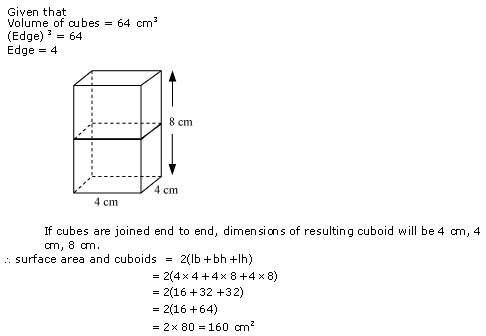Question 2.
A vessel is in the form of a hollow hemisphere mounted by a hollow cylinder. The diameter of the hemisphere is 14 cm and the total height of the vessel is 13 cm. Find the inner surface area of the vessel.

Solution: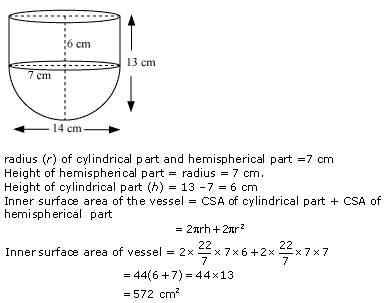Question 3.
A toy is in the form of a cone of radius 3.5 cm mounted on a hemisphere of same radius. The total height of the toy is 15.5 cm. Find the total surface area of the toy.

Solution: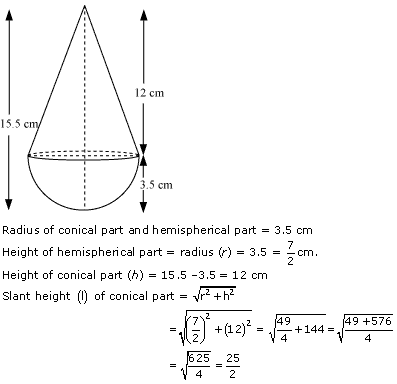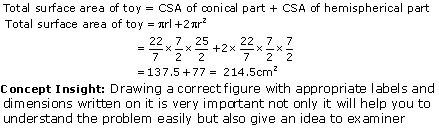Question 4.
A cubical block of side 7 cm is surmounted by a hemisphere. What is the greatest diameter the hemisphere can have? Find the surface area of the solid.

Solution: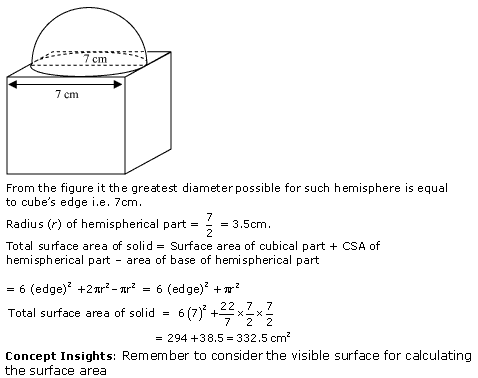Question 5.
A hemispherical depression is cut out from one face of a cubical wooden block such that the diameter d of the hemisphere is equal to the edge of the cube. Determine the surface area of the remaining solid.

Solution: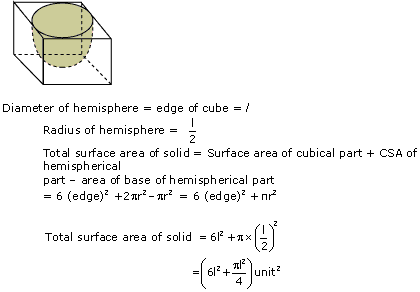Question 6.
A medicine capsule is in the shape of a cylinder with two hemispheres stuck to each of its ends. The length of the entire capsule is 14 mm and the diameter of the capsule is 5 mm. Find its surface area.

Solution: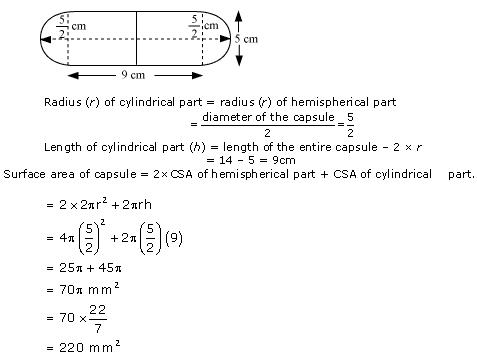Question 7.
A tent is in the shape of a cylinder surmounted by a conical top. If the height and diameter of the cylindrical part are 2.1 m and 4 m respectively, and the slant height of the top is 2.8 m, find the area of the canvas used for making the tent. Also, find the cost of the canvas of the tent at the rate of Rs 500 per m2. (Note that the base of the tent will not be covered with canvas.)

Solution: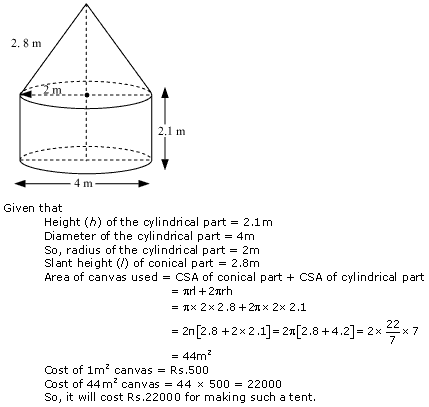Question 8.
From a solid cylinder whose height is 2.4 cm and diameter 1.4 cm, a conical cavity of the same height and same diameter is hollowed out. Find the total surface area of the remaining solid to the nearest cm2.

Solution: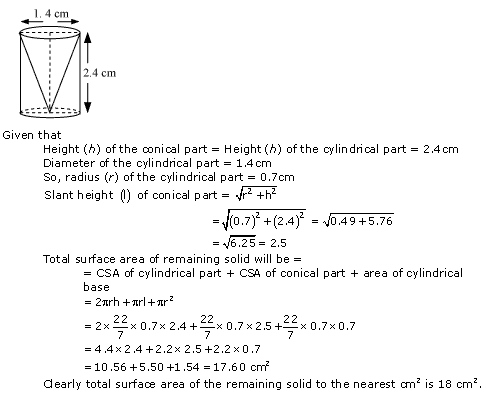Question 9.
A wooden article was made by scooping out a hemisphere from each end of a solid cylinder, as shown in figure. If the height of the cylinder is 10 cm, and its base is of radius 3.5 cm, find the total surface area of the article.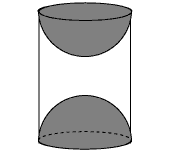Solution: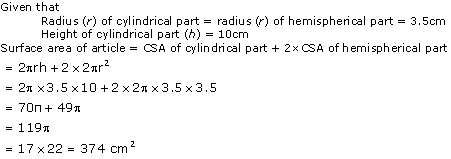We hope the NCERT Solutions for Class 10 Maths Chapter 13 Surface Areas and Volumes Ex 13.1 help you. If you have any query regarding NCERT Solutions for Class 10 Maths Chapter 13 Surface Areas and Volumes Ex 13.1, drop a comment below and we will get back to you at the earliest.